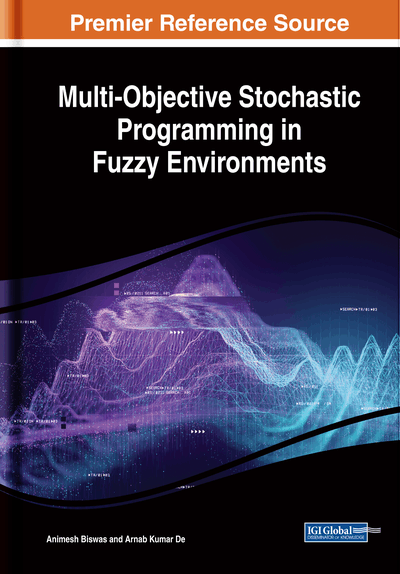# On Solving Fuzzy Multi-Objective Multi-Choice Stochastic Transportation Problems

DOI: 10.4018/978-1-5225-8301-1.ch009
Available
\$33.75
List Price: \$37.50
10% Discount:-\$3.75
TOTAL SAVINGS: \$3.75

## Abstract

This chapter presents solution procedures for solving unbalanced multi-objective multi-choice stochastic transportation problems in a hybrid fuzzy uncertain environment. In this chapter, various types of unbalanced multi-objective fuzzy stochastic transportation models are considered with the assumption that the parameters representing supplies of the products at the origins and demands of the products at the destinations, capacity of the conveyances, associated with the system constraints are either fuzzy numbers (FNs) or fuzzy random variables (FRVs) with some known continuous fuzzy probability distributions. The multi-choice cost parameters are considered as FNs. In this chapter, two objectives are considered: total transportation cost and total transportation time. As the transportation cost mainly depends on fuel prices and since fuel prices are highly fluctuating, the cost parameters are taken as multi-choice cost parameters with possibilistic uncertain nature. The time of transportation mainly depends on vehicle conditions, quality of roads, and road congestion. Due to these uncertain natures, the parameters representing time of transportation are also taken as fuzzy uncertain multi-choice parameters. In this transportation model, these objectives are minimized satisfying the constraints: product availability constraints, requirement of the product constraints, and capacity of the conveyance constraints. Numerical examples are provided for the sake of illustration of the methodology presented in this chapter, and also achieved solutions are compared with the solutions obtained by some existing methodologies to establish its effectiveness.
Chapter Preview
Top

## 9.1 Multi-Objective Multi-Choice Transportation Problem

Each organization always keeps a motivation to find a better process to produce their products and deliver them to the markets in a smooth way. Transportation model is the most powerful frame for fulfilling each goal of the organizations. The transportation problem is a distribution-type linear programming problem (LPP), concerned with transferring goods between various origins and destinations. Generally, in every manufacturing organization two types of transportations are needed. First of all raw materials are transported to the factory and secondly the product produced in the factory are transported from factory to various markets. Therefore, it is the essential part of every organization. Again, the products manufactured in the factory are transferred from factory to various markets in various routes. Therefore, it is an important task for the managers of the organizations to identify exactly one among the various routes in such a manner that the combination of choices should minimize the overall transportation cost.

The basic transportation problem (TP) originally modelled by Hitchcock (1941) and discussed in details by Koopmans (1949). As the coefficient of the objective functions of the TPs could represent the transportation cost, transportation time, loss during transportation, etc. Therefore, in practical life TP is multi-objective in nature. Lee et al. (1973) developed a technique to solve the multi-objective TPs. Diaz (1978) presented an alternative procedure to generate all non-dominated solutions to the multi-objective TPs. Isermann (1979) and Diaz (1979) developed algorithms for identifying all of the non-dominated solutions for a linear multi-objective TP. Ravindran et al., (1987) and Hiller and Leiberman (1990) considered a mathematical model in which an appropriate constraint is to be chosen using binary variables. A method for modeling the multi-choice programming problem, using binary variables was presented by Chang (2007). A methodology for solving a multi-objective TP with nonlinear cost and multi-choice demand was proposed by Maity and Roy (2015).

In classical TPs, it is assumed that the decision maker (DM) is sure about the precise value of availability and demand of the products. In real life, the demand and supply values are not always known precisely due to some uncontrollable factors, insufficient data, fluctuating financial market etc. The probability theory and fuzzy set theory are the two useful techniques to analyze the uncertain situation.

Dantzig (1963) was first to formulate the mathematical model of probabilistic programming where the parameters are random variable with some known probability distribution. The Chance constrained programming (CCP) technique was first introduced by Charnes and Cooper (1959) to solve stochastic programming problems. It was further studied by Vajda (1972), Sengupta (1972) and Luhandjula (1983). Bit et al. (1992) solved the chance constrained multi-objective TP by applying fuzzy programming technique where the parameters are normally distributed random variables. In 2010 Mahapatra et al. (2010) presented a technique to solve multi-objective TP involving log-normal and extreme value distribution, respectively. Mahapatra et al., (2013) proposed a method for solving multi-choice stochastic TP with availability and demand follows extreme value distributed random variables. Quddoos et al., (2014) developed a methodology for solving multi choice stochastic TP with general form of distributions. Recently, Gupta et al. (2018) discussed a solution procedure of a multi-objective capacitated TP in an uncertain environment in which the demand and supply parameters of the problem follows Pareto, Weibull, normal, extreme value, Cauchy and logistic distributed random variables.

## Complete Chapter List

Search this Book:
Reset